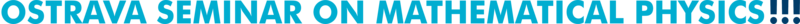Past talks (2017)
 13. February 14, 2017 Roman Popovych (Wolfgang Pauli Institute, Vienna) Point-transformation structures on classes of differential equations Abstract: We discuss various structures constituted by point (resp. contact) transformations on several underlying spaces, which are related to a class of differential equations. This includes the equivalence groupoid of the class, its usual (resp. generalized, resp. effective generalized, resp. extended generalized) equivalence group, point symmetry groups of single equations from the class and infinitesimal counterparts of these objects. Transformational properties of classes of differential equations are characterized in terms of the notion of normalization and its modifications. Thus, a class of differential equations is called normalized if its equivalence group generates its equivalence groupoid. We present a procedure for finding equivalence groupoids. The description of an equivalence groupoid may involve generalizations of equivalence group, partition into normalized subclasses, mappings between classes, etc. The framework developed allows us to revise and to essentially enhance the algebraic method of group classification of differential equations. Slides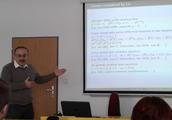14. February 14, 2017 Andrii Khrabustovskyi (Karlsruhe Institute of Technology) Laplace operator in domains with many holes: an overview Abstract: There is well-known classical problem in spectral theory: to investigate how the eigenvalues of the Laplace operator in some fixed domain change if we cut a lot of holes in this domain out (see, e.g., [M. Kac, Rocky Mountain J. Math. 4 (1974)] or [J. Rauch, M. Taylor, J. Funct. Anal. 18 (1975)]). In this talk we present an overview of the results for the Laplace operator with Dirichlet boundary conditions. Some recent results will be discussed as well. Slides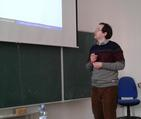15. March 3, 2017 Artur Sergyeyev (Silesian University in Opava) Integrable systems in four independent variables: a new construction based on contact geometry Abstract: We present a novel systematic construction for integrable (3+1)-dimensional dispersionless systems using nonisospectral Lax pairs written in terms of contact vector fields. In particular, we show that, modulo a change of variables, to any pair of rational functions of variable spectral parameter in general position there corresponds a (3+1)-dimensional integrable dispersionless system. 16. March 29, 2017 Čestmír Burdík (Czech Technical University in Prague) Algebraic Bethe ansatz Abstract: On the example of gl(2) we will show the formulation of algebraic Bethe ansatz. The generalization to nested Bethe ansatz for the gl(3) case will be done. Finally some new results will be mentioned.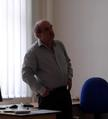17. April 4, 2017 Andrey Krutov (Institute of Mathematics, Polish Academy of Sciences) Lie algebroids over infinite jet spaces (Joint work with A.V. Kiselev) Abstract: We define Lie algebroids over infinite jet spaces. The example of such construction is given by Hamiltonian operators and Lie algebra-valued zero-curvature representations for partial differential equations. The talk is based on the following papers: (1) A.V. Kiselev, J.W. van de Leur, Variational Lie algebroids and homological evolutionary vector fields, Theor. Math. Phys. 167 (2011), no.3, 772-784; arXiv:1006.4227. (2) A.V. Kiselev, A.O. Krutov, Non-Abelian Lie algebroids over jet spaces, J. Nonlin. Math. Phys. 21 (2014), no.2, 188-213; arXiv:1305.4598. Slides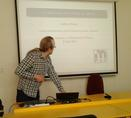18. April 6, 2017 Andrey Krutov (Institute of Mathematics, Polish Academy of Sciences) Gardner's deformations of integrable systems (Joint work with A.V. Kiselev) Abstract: By tracking the relations between zero-curvature representations (ZCR) and Gardner's deformations, we construct the solution of the deformation problem for the N=2, a=4 SKdV equation. We show that the ZCR found by Das et al. yields a system of new nonlocal variables such that their derivatives contain the Gardner deformation for the classical KdV equation. In turn, from this system of nonlocalities we derive Gardner's deformation for N=2 supersymmetric a=4 Korteweg-de Vries equation. Likewise we obtain Gardner's deformation for the Krasil'shchik-Kersten system from a zero-curvature representation found for it by Karasu-Kalkanli et al.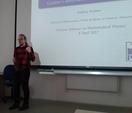19. April 7, 2017 Andrey Krutov (Institute of Mathematics, Polish Academy of Sciences) On gradings modulo 2 of simple Lie algebras in characteristic 2 (Joint work with A. Lebedev) Abstract: In characteristic 2, the classification of modulo 2 gradings of simple Lie algebras is vital for the classification of simple finite dimensional Lie superalgebras: with each grading a simple Lie superalgebra is associated, see arXiv:1407.1695 (S. Bouarroudj, A. Lebedev, D. Leites, and I. Shchepochkina, "Restricted Lie (super)algebras, classification of simple Lie superalgebras in characteristic 2"). No classification of gradings was known for any type of simple Lie algebras, bar restricted Zassenhaus algebras (a.k.a. Witt algebras, i.e., Lie algebras of vector fields with truncated polynomials as coefficients) on not less than 3 indeterminates. Here we completely describe gradings modulo 2 for several series of simple Lie algebras: special linear, two inequivalent orthogonal, and projectivizations of their derived, except for psl(4) for which a conjecture is given. All of the corresponding superizations are known, but a corollary proves non-triviality of a deformation of a simple Lie superalgebra (new result). For nonrestricted Zassenhaus algebras on one indeterminate, there are more types of modulo 2 gradings than for the restricted Zassenhaus algebras, and several corresponding simple Lie superalgebras seem to be new. This is proved for small values of the height of divided powers; the general answer is conjectural.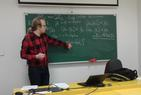20. April 12, 2017 Radosław Kycia (Krakow University of Technology and Masaryk University, Brno) Singularities, self-similar solutions and their generalizations Abstract: I will present our recent results on self-similar solutions of semilinear wave equations, their generalizations and related problems involving (movable and fixed) singularities of differential equations. Bibliography:  R. Kycia, G. Filipuk, On the generalized Emden-Fowler and isothermal spheres equations.  R. Kycia, G. Filipuk, On the singularities of the Emden-Fowler type equations.  R. Kycia, On similarity in the evolution of semilinear wave and Klein-Gordon equations: Numerical surveys.  R. Kycia, On self-similar solutions of semilinear wave equations in higher space dimensions. Slides Movies (simulations were obtained using moving mesh method with adaptive timestepping):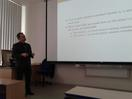21. May 15, 2017 Adam Hlaváč (Silesian University in Opava) On the constant astigmatism equation and surfaces of constant astigmatism Abstract   Slides 22. June 13, 2017 Mirosław Lachowicz (University of Warsaw) Topological chaos and some applications Abstract: It is known that the chaotic behavior can occur in some infinite-dimensional linear systems. I am going to discuss the notion of topological chaos with application to a class of infinite system of linear ODEs (with variable coefficients) describing the population of cancer cells divided into sub-populations characterized by different levels of resistance to antineoplastic drugs.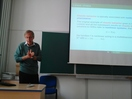23. June 13, 2017 David Saunders (University of Ostrava) Lagrangians with reduced-order Euler-Lagrange equations Abstract: Any Lagrangian form of order k obtained by horizontalization of a form of order k-1 gives rise to Euler-Lagrange equations of order strictly less than 2k. But these are not the only possibilities. For example, with two independent variables, the horizontalization of a first-order 2-form gives a Lagrangian quadratic in the second-order variables; but there are also cubic second-order Lagrangians with third-order Euler-Lagrange equations. In this talk I shall show first that any Lagrangian of order k with Euler-Lagrange equations of order less than 2k must be a polynomial in the k-th order variables of order not greater than the number of different symmetric multi-indices of length k. I shall then describe a geometrical construction, based on Peter Olver's idea of differential hyperforms, which gives rise to Lagrangians with reduced-order Euler-Lagrange equations. I believe (and might be able to prove, though this is not guaranteed!) that every such Lagrangian arises in this way. Slides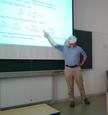24. June 14, 2017 Waldemar Hołubowski (Silesian University of Technology, Gliwice, Poland) Groups, rings and Lie algebras of infinite matrices Abstract: Our talk is devoted to the algebraic study of groups, rings and Lie algebras of infinite matrices over associative rings. Such infinite matrices arise naturally in purely algebraic research, both in their own right, but also in connection with construction of examples and counter-examples in group theory, ring theory. However, historically, the main motivation to study infinite matrices came from their numerous spectacular applications to classical analysis, harmonic analysis, functional analysis, combinatorics, mathematical physics, probability theory. We will survey some new results obtained for Lie algebras.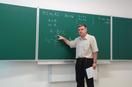25. June 26, 2017 Matěj Tušek (Czech Technical University in Prague) A geometric Iwatsuka type effect in quantum layers Abstract: We consider magnetic Dirichlet Laplacian on a layer of a fixed width constructed along a two-dimensional hypersurface. The magnetic field will always be uniform, but the geometry of the layer may be non-trivial. If the layer is planar and the field is perpendicular to it the spectrum consists of infinitely degenerate eigenvalues. We will study translationally invariant geometric perturbations and derive several sufficient conditions under which the spectrum, in its entirety or a part of it, becomes absolutely continuous. The talk is based on a joint work with T. Kalvoda and P. Exner. Slides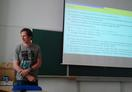26. September 19, 2017 Martin Markl (Institute of Mathematics, Czech Academy of Sciences, Prague) Open-closed modular operads, Cardy condition and string field theory Abstract: We prove that the modular operad of diffeomorphism classes of Riemann surfaces with both 'open' and 'closed' boundary components, in the sense of string field theory, is the modular completion of its genus 0 part quotiented by the Cardy condition. We also provide a finitary presentation of a version of this modular two-colored operad and characterize its algebras via morphisms of Frobenius algebras.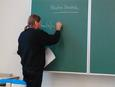27. October 3, 2017 Dardo Goyeneche (Jagiellonian University in Krakow) Quantum combinatorial designs Abstract: We present a comprehensive introduction to quantum orthogonal arrays as a natural generalization of orthogonal arrays to quantum mechanics. These quantum combinatorial designs naturally induce the concepts of quantum Latin squares, cubes, and hypercubes in general, and a notion of orthogonality. We derive a simple construction of quantum orthogonal arrays composed by an arbitrary number of columns. Furthermore, we show how to use quantum orthogonal arrays to construct absolutely maximally entangled states for multipartite quantum systems. [No notion about quantum mechanics is required to understand the seminar]. Reference: arXiv:1708.05946. Slides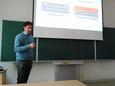28. October 4, 2017 Anna Lytova (University of Opole, Poland) On the CLT for spectral statistics of Wigner and sample covariance random matrices Abstract   Slides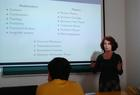29. October 17, 2017 Arman Taghavi-Chabert (University of Torino) Twistor geometry of null foliations Abstract: We give a description of local null foliations on an odd-dimensional complex quadric Q in terms of complex submanifolds of its twistor space defined to be the space of all linear subspaces of Q of maximal dimension.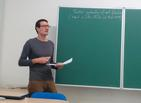30. October 24, 2017 Dietrich Burde (University of Vienna) Geometric structures on Lie groups and post-Lie algebras Abstract   Slides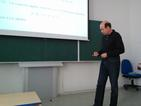31. October 25, 2017 Geoff Prince (Australian Mathematical Sciences Institute, Melbourne) Variationality of PDEs Abstract: When can a system of PDEs be expressed in variational form? And what can we say about the uniqueness of such variational forms? I will import some concepts from the ODE case to frame the problem and make some conjectures. This is joint work with Olga Rossi and Thoan Do. Slides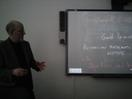32. November 2, 2017 Oleg Morozov (AGH University of Science and Technology, Krakow) Deformations and extensions of infinite-dimensional Lie algebras, exotic cohomology, and integrable nonlinear partial differential equations Abstract: The important unsolved problem in theory of integrable systems is to find conditions guaranteeing existence of a Lax representation for a given PDE. The exotic cohomology of the symmetry algebras opens a way to formulate such conditions in internal terms of the PDEs under the study. The talk will discuss the recent developments of this approach. I will consider examples of infinite-dimensional Lie algebras with nontrivial second exotic cohomology groups and show that the Maurer-Cartan forms of the associated extensions of these Lie algebras generate Lax representations for integrable systems, both known and new ones. Slides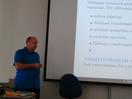33. November 14, 2017 Robert Wolak (Jagiellonian University in Krakow) Hessian manifolds and foliations and their application in Information Geometry Abstract: We will present an introduction to the geometry of Hessian manifolds and foliations in view of applications to Information Geometry. References: 1. S-I. Amari and H. Nagaoka, Methods of Information Geometry, AMS & Oxford Univ. Press, 2000. 2. H. Shima, The Geometry of Hessian Structures, World Scientific, 2007. 3. M. Nguiffo Boyom and R. Wolak, Transversely Hessian foliations and information geometry, Intern. J. Math. 27 (2016), no.11, 1650092. Slides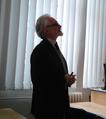34. December 5, 2017    Vladimir Lotoreichik (Nuclear Physics Institute, Czech Academy of Sciences) Spectral optimization for the Robin Laplacian on exterior domains Abstract   Slides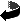Ostrava Mathematical Seminar
Created: Mon Mar 20 2017